# Project 2: Filter (Algo Answers)

You can find the handout for this project here. Please read the handout before working on this algo assignment!

## Convolution

### Discrete Convolution

You are given the following 1D kernel and input image. Assume that any pixel outside the bounds of the input image has value 0 (i.e. the image is zero-padded).

What is the output image when the kernel and image are convolved with each other? Present your answer as a vector of size 6, representing the output image.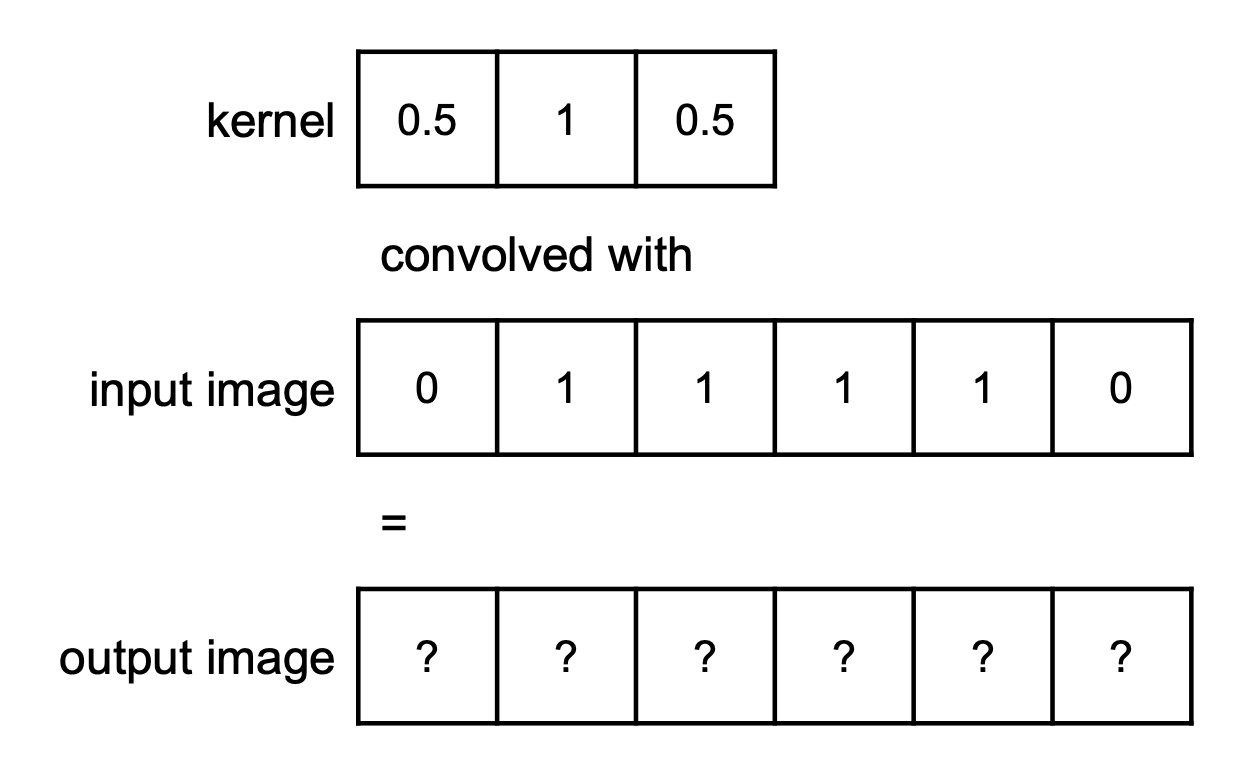Figure 1: 1D arrays representing the input image, kernel, and output image (unfilled).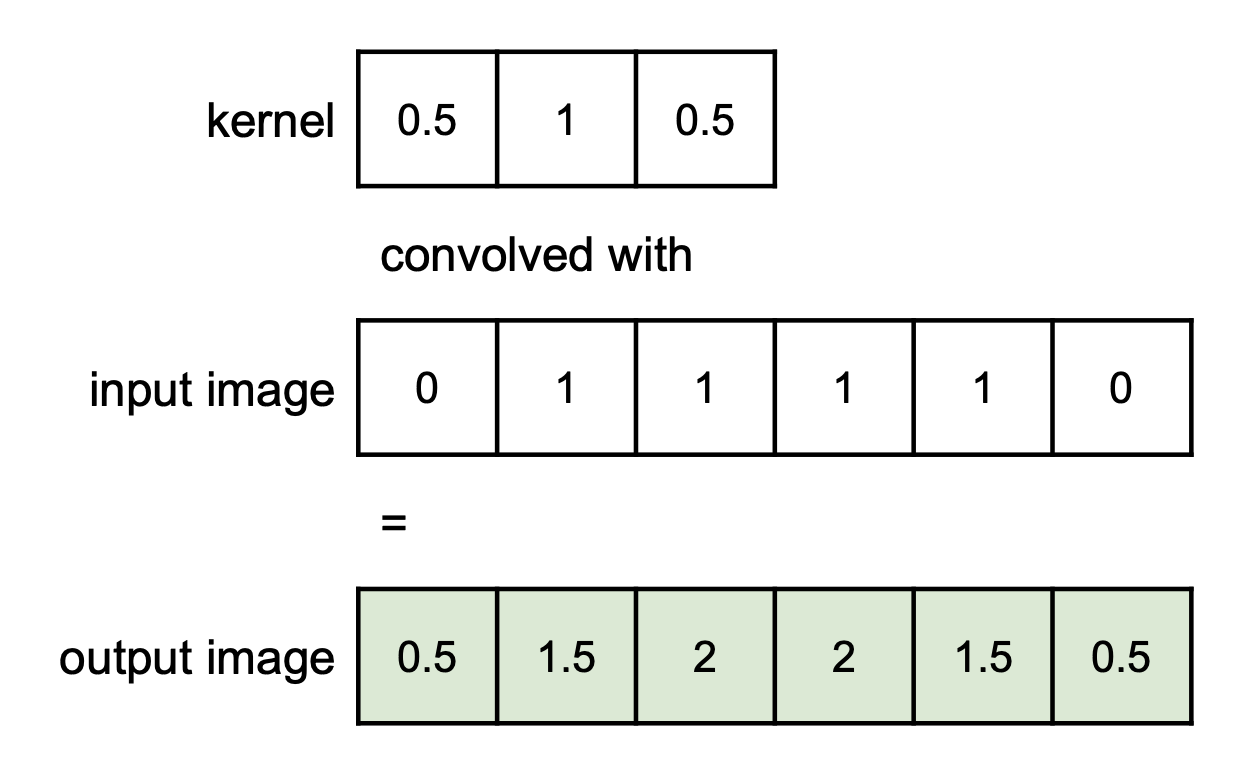Figure 1 (ans): 1D arrays representing the input image, kernel, and output image (filled).

Explanation:

The value of the output image at index is given by the following inner product:

Applying this formula for each index results in the image above.

### Continuous Convolution

Convolve the below function and filter. What is the value of at in the output?Figure 2: Image as a continuous signal with filter function for Q1.2

. See the next question for the output of the convolution.

### Visualizing Convolution

Draw the shape of the output function obtained in the previous question (figure 2).

Explanation:

Imagine the filter, flipped (such that becomes ), sliding horizontally across the function.

While doing this, we can identify 4 important points we need to accurately graph the output function:

• The first point where the boxes start to overlap (),
• The first point where the boxes fully overlap (),
• The point where the boxes stop fully overlapping (), and
• The point where the boxes stop overlapping at all. (),

As the maximum overlapping area of both inputs is , the maximum value of the output must also be .

From to the output ramps up from to linearly. Then from to the output is constant at . Finally from to the output once again ramps linearly down to . Everywhere else, the output is .

## Blurring

Let's try to generalize our solution to the earlier discrete convolution problem.

Consider a 1D kernel of radius that represents a blurring filter. We apply this blur (via convolution) to an input image resulting in a new image .

All of , , and can be considered as 1D arrays storing intensities where gives the intensity of the -th pixel. Remember that the kernel is zero-indexed so gives the left-most value in the kernel array.

We are interested in writing out this convolution as summation over the values of and . For some index within the bounds of the image, please write out the summation equation for in terms of , , , and .

You may ignore edge effects for the purposes of this question (i.e. feel free to "incorrectly" index out-of-bounds).

Explanation:

Remember that we must define a summation over the element-wise multiplication of function and kernel , using the kernel's elements .

We must also ensure that is correctly "lined up with" .

With these two constraints in hand, we are ready to define a summation either from to , or from to with different offsets.

## Scaling

### Scaling Up

What is the filter support width when you scale by a factor of , where ?

Explanation:

For this explanation, please assume a 1D image.

A triangle filter of width (in units of pixels in the original image) guarantees that, for each output pixel, we are sampling two* pixels in the original image. This effectively performs linear interpolation between the two pixels, and when scaling images, this is usually the way to go (in 2D, it is known as bilinear interpolation).

* Technically...

There are cases where, for an output pixel, we sample only "one" pixel in the original image:

• An output pixel at the edge of the image samples only the input pixel at the exact same position, and its neighbor pixel (but with weight 0, since it's at the tip of the triangle);
• In fact, any output pixel which has the exact same position as an input pixel will sample only that input pixel, plus its neighbors (again, with weight 0).
• This makes sense, since we already know what color that pixel should be: the same color as the input pixel at the same position.

### Scaling Down

What is the filter support width when you scale by a factor of , where ?

Explanation:

The reason we use a filter while scaling is to avoid aliasing. Ideally, we'd like to use an ideal low-pass filter: this would be a box in the frequency domain, and a sinc in the spatial domain. Of course, we cannot use a sinc, so we approximate this sinc with a triangle (for scaling, in CS 1230).

This approximation is what gives rise to the answer . It can be found in the image processing lecture slides.

Extra: but why is it ?

It's not necessary for you to understand the math behind this derivation, but given that we have an input image with some max frequency such that (i.e. it obeys the Nyquist limit), we want an output image with max frequency such that:

This corresponds to a filter which looks like a box function with width in the frequency domain. Via an inverse Fourier transform (or simply by Googling it), we can find that its dual in the spatial domain looks like a sinc which first intersects at .

We approximate this sinc with a triangle with width .

### Naïve Back-mapping

Recall that back-mapping refers to finding the correct filter placement given a pixel in the output image.

The naïve back-map function is , but the correct back-map function is .

Suppose we wanted to scale down a 9-pixel 1D image by a factor of ().

Draw a picture to show where we would sample the original image using the naïve back-map function. Please include an illustration of the filter above each sample point.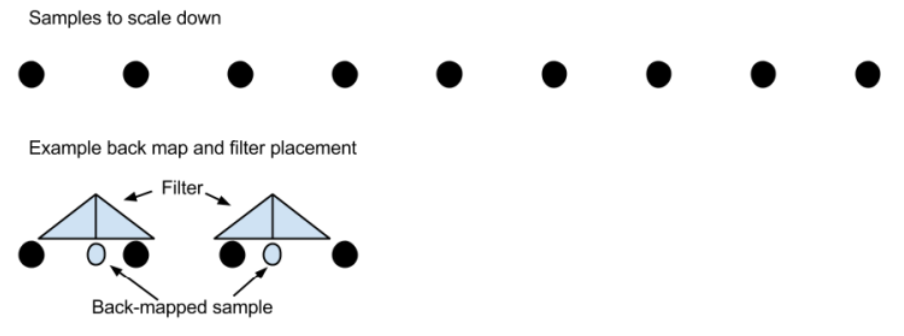Figure 3: Example of how to draw the sample points with the filter.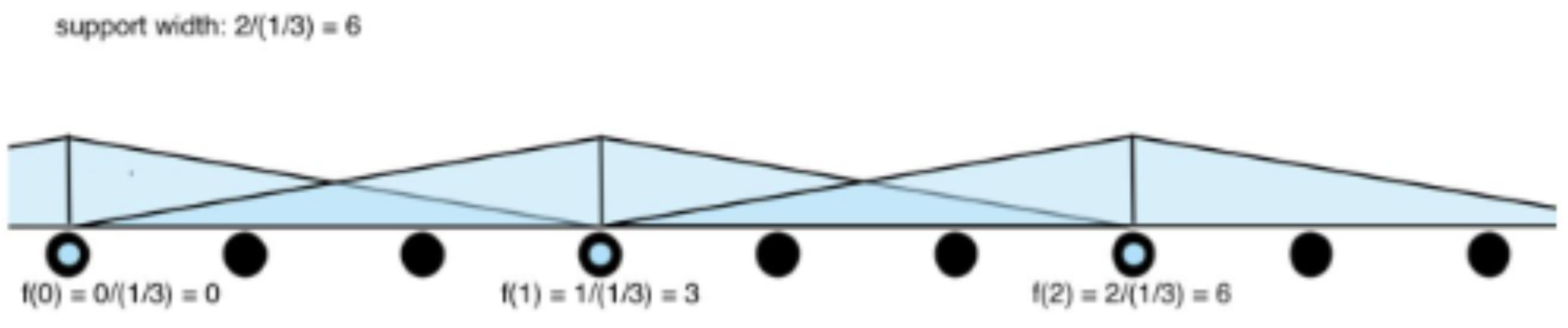Figure 3 (ans 1): Example of how to draw the sample points with the filter.

Explanation:

Since we are scaling the image by a factor of , the support width of the filter should be 6. The back-mapping function tells us where to place the filter. For the index of the new image, we need to place our filter at index of the original image. Using the same logic, we place our filter at index 3 and 6 of the original image.

If you got both this question and the next question correct, you got an extra point on the algo assignment!

### Correct Back-map

Draw another picture to show where we would sample the original image using the naïve back-map function. Again, please include an illustration of the filter above each sample point.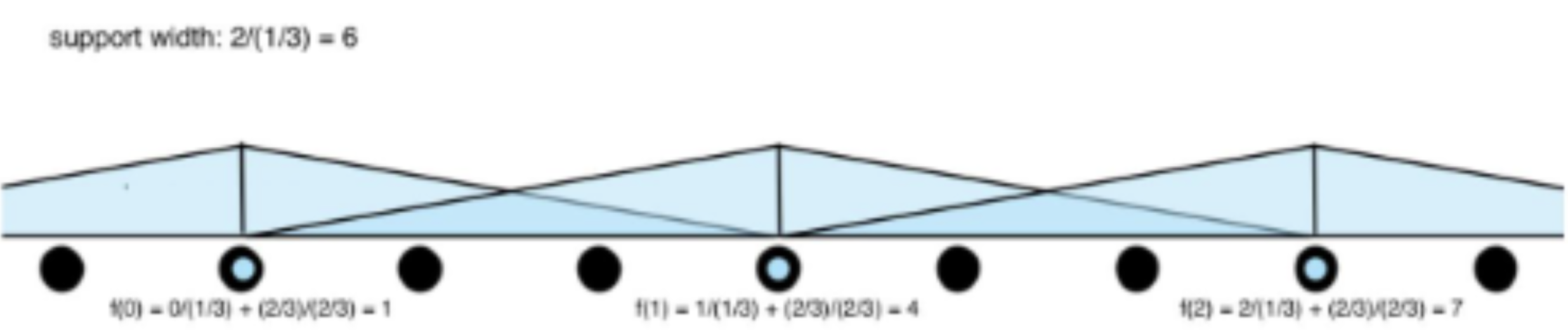Figure 3 (ans-2): Example of how to draw the sample points with the filter.

Explanation:

Again, the support width of our filter should be 6 because we are scaling the image by a factor of . The difference between the naïve and correct back-maps is where we place our filter. Using the correct back-map function, we get: index 0 of the new image maps to index 1 of the original image, index 1 of the new image maps to index 4 of the original image, and index 2 of the new image maps to index 7 of the original image.

If you got both this question and the previous question correct, you got an extra point on the algo assignment!

## Duality of Domains

### Visualizing Sinc and Box Duals

Take a look at the box function in figure 4 (left) below. Its dual in the frequency domain is the sinc function, in figure 4 (right). Note that there are no labels on the axes, by design.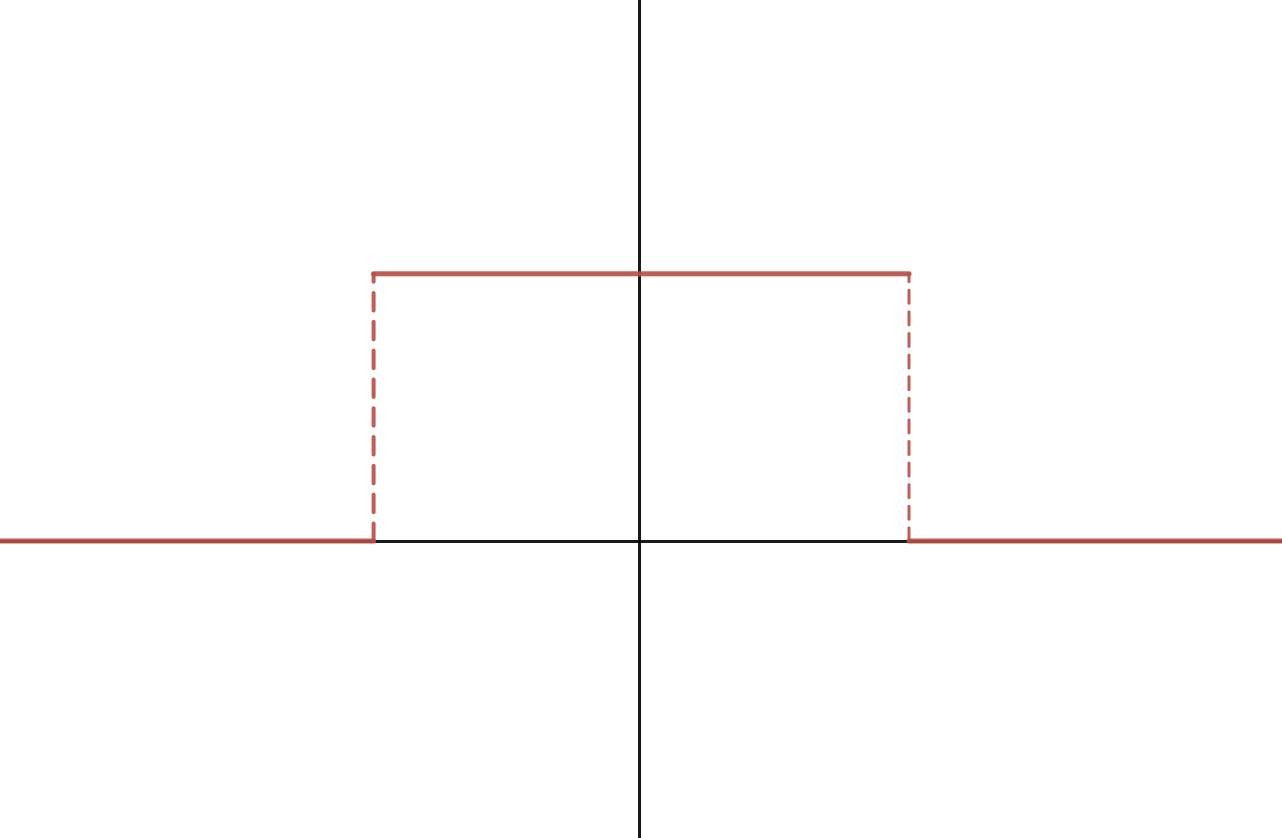Figure 4: A box function with its dual, a sinc function.

Please sketch the dual of the box function in figure 5. Your drawing doesn't need to be perfectly accurate; we just need to see that you get the idea.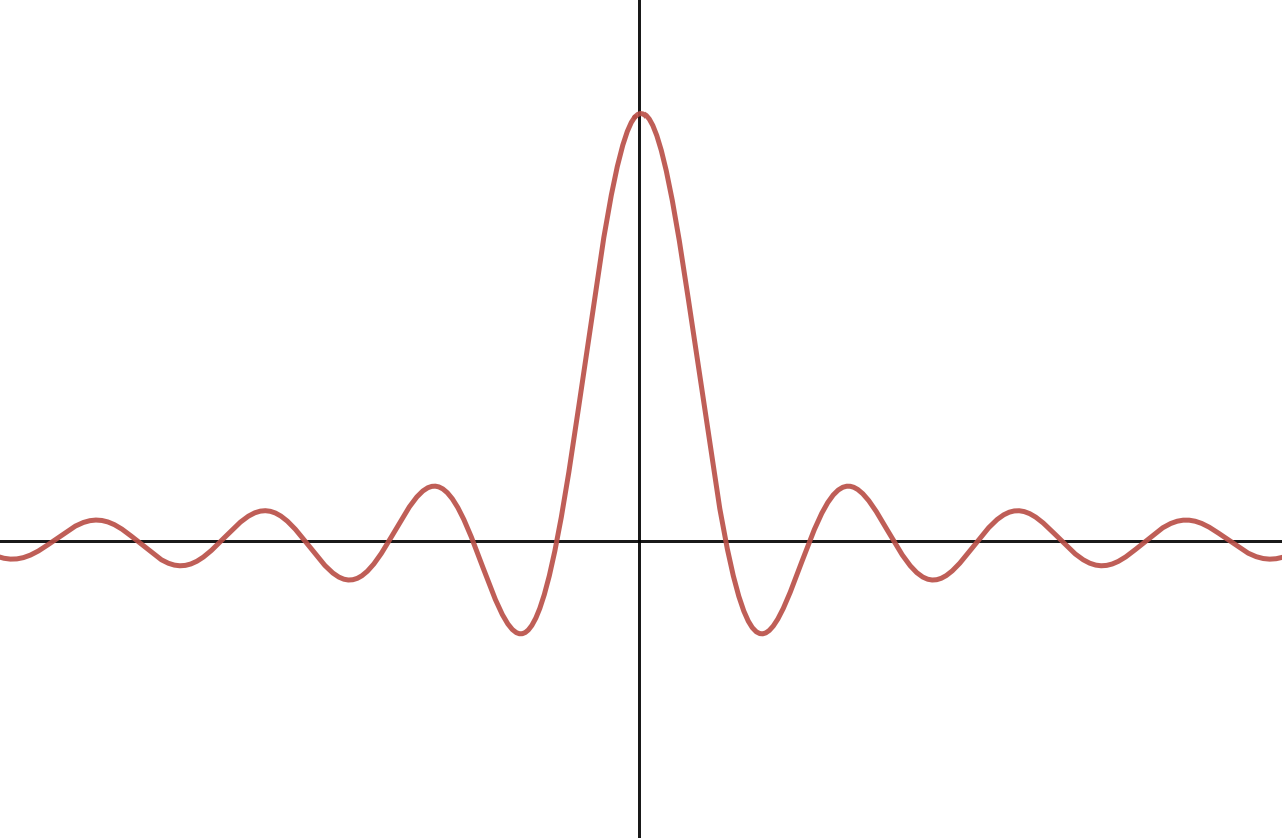Figure 5 (ans): The dual of the wider box function, a sinc which is about half as narrow as before.

Explanation:

A wider box function in the frequency domain means that there are more signals with higher frequency. To reflect this fact in the spatial domain, the sinc function needs to have higher frequency, or, oscillate more. Visually, the sinc function becomes narrower.

### Approximating Sinc

What do we usually use to approximate the sinc function, and why do we have to make this approximation when translating these theoretical concepts into code?

A Gaussian function. Though, in cases where the approximation doesn't have to be as good, a triangle function can be used instead.

Explanation:

Both the Gaussian and triangle filters are cheaper to use.

Sinc functions are infinite in extent, and it's simply not feasible to perform discrete convolution with an infinitely large, nor would it be performant to approximate it with a "very large" one.

Gaussians are technically also infinite in extent, but fall to almost-zero fairly quickly. Thus, Gaussians can be used as a cheaper approximations to the sinc filter. Compared to the triangle, it also has a nice property: a Gaussian's dual in the frequency domain is another Gaussian, which means it better approximates a good low-pass filter.

### Sampling in the Frequency Domain

If we're sampling a continuous function at a frequency of samples per unit, what is the largest frequency we can represent, according to the Nyquist limit?

Any frequency less than, but not equal to .

Explanation:

In order to capture all frequencies in a signal, we must sample at a rate that is strictly higher than 2 times the highest frequency. Thus, if are sampling samples per unit, we can only represent a continuous function with a frequency of lower than .

### Frequency Plots

Now, examine the following frequency plot of a 1D, continuous signal. If you were going to sample this signal at a rate of 8 samples per unit, to avoid aliasing, you would use what we know about the Nyquist limit to pre-filter it. Sketch the new frequency plot after someone has performed this pre-filtering step optimally.